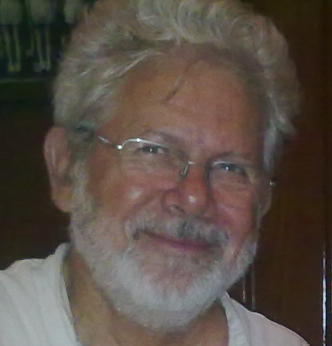# The derived product on a Q-manifold

This went “under my radar” for a while, I am not sure why, but anyway…

Q-manifolds
Recall that a Q-manifold is a supermanifold $$M$$ equipped with a homological vector field, that is an odd vector field $$Q$$ such that $$[Q,Q]=2 Q^{2}=0$$.

The algebra of functions $$C^{\infty}(M)$$ is thus a supercommutative differential algebra. The product of two functions is again a function and importantly this is supercommutative:

$$f g = (-1)^{\widetilde{f} \widetilde{g} } g f$$,

where I denote the Grassmann parity of a homogeneous function by the “tilde”. Just as on a classical manifold, the product of two functions is associative:

$$f(gh) = (fg)h$$,

for all functions f,g,h. Also important is that the product itself does not carry any Grassmann parity, thus

$$\widetilde{fg} = \widetilde{f} + \widetilde{g}$$.

The prime example here is to take $$M = \Pi TN$$ where $$N$$ is a classical manifold. The functions $$C^{\infty}(\Pi TM)$$ are identified with (pseudo)differential forms and the homological vector field is just the de Rham differential.

The derived product

Definition Let $$(M,Q)$$ be a Q-manifold. Then the derived product on $$C^{\infty}(M)$$ is defined as

$$f \ast g = (-1)^{\widetilde{f}+1} Q(f)g$$.

1. The derived product is Grassmann odd, $$\widetilde{f\ast g} = \widetilde{f} + \widetilde{g}+1$$
2. The derived product is clearly not supercommutative

Remark One can consider a derived product on any differential algebra, one does not need the supercommutivity, but as I am interested in supergeometry the definition here suits well.

Right away the derived product seems a strange beast, it carries Grassmann parity itself. Being noncommutative is also interesting, but we are used to noncommutative forms of multiplication.

Theorem The derived product is associative.

Proof Explicitly
$$f \ast (g \ast h) = (-1)^{\widetilde{f} + \widetilde{g}} Q(f)Q(g)h$$.

Now consider

$$(f \ast g)\ast h = (-1)^{\widetilde{f} + \widetilde{g}}Q ((-1)^{\widetilde{f}+1}Q(f)g )h$$.

Using the fact that $$Q$$ is homological, that is odd and squares to zero we get

$$(f \ast g)\ast h =(-1)^{\widetilde{f} + \widetilde{g}} Q(f)Q(g) h$$.

QED

Theorem $$f \ast g = (-1)^{(\widetilde{f}+1)(\widetilde{g}+1)} g \ast f + (-1)^{\widetilde{f}+1}Q(fg)$$.

Proof Left as an exercise for the readers.

The above theorem shows very explicitly that the derived product is not supercommutative. It is however antisymmetric if and only if $$Q(fg)=0$$.

Where has this come from?
As far as I am aware, the notion of a derived product can be found in the work of Loday;

J.L. Loday. Dialgebras. In Dialgebras and related operads, 7-66, Lecture Notes in Math., 1763, Springer, Berlin 2001.Jean-Louis Loday,
(12 January 1946 – 6 June 2012)

Concluding remarks
We have a kind of noncommutative deformation of the algebra of functions on a Q-manifold via the derived product. This “new product” carries Grassman parity and is associative, it is closer to the standard notion of a product than say a Lie algebra.

I am sure there is lots more to say about derived products, but this is something I am only just beginning to explore. Watch this space…